# MA.912.DP.2.6Export Print
Given a scatter plot with a line of fit and residuals, determine the strength and direction of the correlation. Interpret strength and direction within a real-world context.

### Clarifications

Clarification 1: Instruction focuses on determining the direction by analyzing the slope and informally determining the strength by analyzing the residuals.
General Information
Subject Area: Mathematics (B.E.S.T.)
Strand: Data Analysis and Probability
Status: State Board Approved

## Benchmark Instructional Guide

### Terms from the K-12 Glossary

• Line of Fit
• Numerical Data
• Scatter Plot

### Vertical Alignment

Previous Benchmarks

Next Benchmarks

### Purpose and Instructional Strategies

In grade 8, students informally fitted a line to a scatter plot. In Algebra I, students use the slope and residuals of a line of fit to determine the strength and direction of the correlation. In later courses, students will use the slope and residuals to more quantitatively determine the strength of the correlation.
• A residual is a measure of how well a line predicts an individual data point. It can be illustrated by the vertical distance between a data point and the regression line. Each data point has one residual given by the equation $R$ = $D$$P$, where $R$ represents the residual, $D$ represents the $y$-coordinate of data value and $P$ represents the $y$-coordinate of predicted value. Residuals are positive if data points are above the regression line and negative if data points are below the regression line. If the regression line actually passes through the point, the residual at that point is zero.
• The slope of the line and the residuals determine the sign and strength of the correlation. A line with a positive slope indicates a positive correlation. A line with a negative slope indicates a negative correlation. Residuals with smaller absolute values indicate stronger correlations. Residuals with larger absolute values indicate weaker correlations.
• If the slope is close to 0, then the correlation may be considered weak even when the residuals are all small. A slope near zero indicates that the independent variable has little effect on the dependent variable.
• Instruction focuses on real-world contexts and includes the use of technology.
• A residual plot has the residual values on the vertical axis; the horizontal axis displays the $x$-variable.
• Once residuals are calculated, students can determine the number of positive and negative residuals and the largest and smallest residuals.
• Outliers, which are observed data points that are far from a line of fit, can be determined from the residual as points whose residuals have a large absolute value.

### Common Misconceptions or Errors

• Students may not be able to distinguish between a scatter plot and a residual graph.
• Students may forget that residual graphs consist of the ordered pair: independent, residual.
• Students may not be able to determine an appropriate model (linear/nonlinear) from a residual graph.
• Students may think that a correlation is strong when the residuals are small and the slope is close to zero.

### Strategies to Support Tiered Instruction

• Teacher co-creates an anchor chart that provides examples of residual graphs for linear models.
• Instruction includes the opportunity to use colors to identify the $x$- and $y$-values in the original data and the $x$- and $y$-values in the ordered pairs associated with the residual graph, and highlight the $x$- and $y$-axis of the residual graph in the same color in order to see the relationship.
• For example, for the data point (6, −0.5), the residual point is (6, 0.85) based on the line fit being $y$ = −0.6$x$ + 2.25.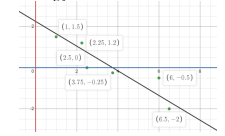Instructional Task 1 (MTR.3.1, MTR.4.1, MTR.7.1
• Crickets are one of nature’s more interesting insects, partly because of their musical ability. In England, the chirping or singing of a cricket was once considered to be a sign of good luck. Crickets will not chirp if the temperature is below 40 degrees Fahrenheit (°F) or above 100 degrees Fahrenheit (°F). A scatter plot is shown with the line of best fit, which can be described by the model $y$ = 0.214$x$ + 32.317.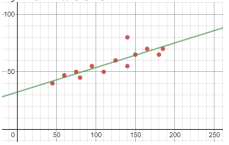• The residuals ($r$) based on the scatter plot are shown.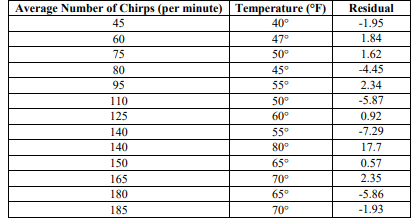• Part A. Determine if the data has a positive or negative correlation.
• Part B. Determine the strength of the correlation.
• Part C. Compare your answers from Part A and B with a partner.
• Part D. Do you notice any possible outliers? Do they effect the judgment of the strength of correlation from Part B?

### Instructional Items

Instructional Item 1
• Based on the data, we know that the line of best fit for the relationship between fat grams and the total calories in fast food using the given data below can be represented by $y$ = 11.44$x$ + 219.89. The residuals of this data have been calculated and are represented in the last column.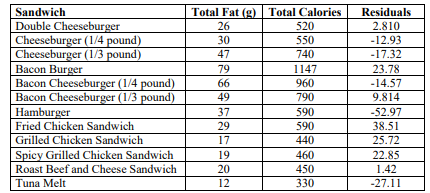• All of this data is represented on the scatter plot.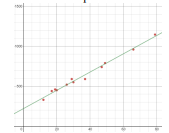• Based on this scatter plot and the residuals, interpret the strength and direction of the correlation of the line of fit as it relates to total grams of fat and total calories of fast food.

*The strategies, tasks and items included in the B1G-M are examples and should not be considered comprehensive.

## Related Courses

This benchmark is part of these courses.
1200310: Algebra 1 (Specifically in versions: 2014 - 2015, 2015 - 2022, 2022 and beyond (current))
1200320: Algebra 1 Honors (Specifically in versions: 2014 - 2015, 2015 - 2022, 2022 and beyond (current))
1200370: Algebra 1-A (Specifically in versions: 2014 - 2015, 2015 - 2022, 2022 and beyond (current))
1200400: Foundational Skills in Mathematics 9-12 (Specifically in versions: 2014 - 2015, 2015 - 2022, 2022 and beyond (current))
1210300: Probability and Statistics Honors (Specifically in versions: 2014 - 2015, 2015 - 2019, 2019 - 2022, 2022 and beyond (current))
7912080: Access Algebra 1A (Specifically in versions: 2014 - 2015, 2015 - 2018, 2018 - 2019, 2019 - 2022, 2022 and beyond (current))
1200315: Algebra 1 for Credit Recovery (Specifically in versions: 2014 - 2015, 2015 - 2022, 2022 and beyond (current))
1200375: Algebra 1-A for Credit Recovery (Specifically in versions: 2014 - 2015, 2015 - 2022, 2022 and beyond (current))
7912075: Access Algebra 1 (Specifically in versions: 2014 - 2015, 2015 - 2018, 2018 - 2019, 2019 - 2022, 2022 and beyond (current))
1210305: Mathematics for College Statistics (Specifically in versions: 2022 and beyond (current))

## Related Access Points

Alternate version of this benchmark for students with significant cognitive disabilities.
MA.912.DP.2.AP.6: Given a scatter plot with a line of fit and residuals, determine the strength and direction of the correlation. Interpret strength and direction within a real-world context.

## Related Resources

Vetted resources educators can use to teach the concepts and skills in this benchmark.

## Lesson Plans

Students will explore voter turnout data for three gubernatorial general elections before and after the passage of the 19th Amendment. They will interpret the correlation of raw voter turnout vs. eligible population using a scatterplot, determine its direction by analyzing the slope and informally determine its strength by analyzing the residuals. Students will draw some conclusions and discuss what a correlation means and how it differs from causation in the context of elections in this integrated lesson.

Type: Lesson Plan

Spreading the Vote - Part 2:

Students will explore voter turnout data for three gubernatorial general elections before and after the passage of the 19th Amendment. They will interpret the correlation of eligible population vs. percentage of voter turnout using a scatterplot, determine its direction by analyzing the slope and informally determine its strength by analyzing the residuals. Students will draw some conclusions and discuss what a correlation means and how it differs from causation in the context of elections in this integrated lesson.

Type: Lesson Plan

Scatter plots, spaghetti, and predicting the future:

Students will construct a scatter plot from given data. They will identify the correlation, sketch an approximate line of fit, and determine an equation for the line of fit. They will explain the meaning of the slope and y-intercept in the context of the data and use the line of fit to interpolate and extrapolate values.

Type: Lesson Plan

## Perspectives Video: Professional/Enthusiast

Analyzing Wildlife Data Trends with Regression :

Dr. Bill McShea from the Smithsonian Institution discusses how regression analysis helps in his research.

This video was created in collaboration with the Okaloosa County SCIENCE Partnership, including the Smithsonian Institution and Harvard University.

Type: Perspectives Video: Professional/Enthusiast

## Student Resources

Vetted resources students can use to learn the concepts and skills in this benchmark.

## Parent Resources

Vetted resources caregivers can use to help students learn the concepts and skills in this benchmark.